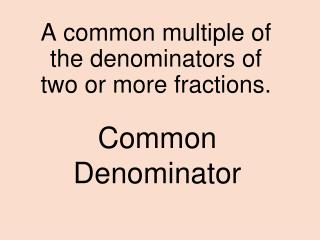DownloadDownload PresentationCommon Denominator

# Common Denominator

Télécharger la présentation## Common Denominator

- - - - - - - - - - - - - - - - - - - - - - - - - - - E N D - - - - - - - - - - - - - - - - - - - - - - - - - - -
##### Presentation Transcript

1. Common Denominator A common multiple of the denominators of two or more fractions.

2. Area The measure of the surface enclosed by a 2-dimensional geometric figure.

3. Composite Number A whole number greater than 1 that has more than two factors.

4. In repeating decimals, the line or bar placed over the digits that repeat. . Bar notation

5. Equivalent fractions Fractions that have the same value.

6. Terminating Decimal A decimal whose digits end.

7. Factor Tree A diagram showing the prime factorization of a number.

8. What order is the Metric System. K H D / D C M

9. Least Common Denominator The lease common multiple of the denominators of two or more fractions.

10. An equation that shows the relationship among certain quantities. Formula

11. A base-ten system of measurement using the base units: meter for length, kilogram for mass, and liter for capacity. Metric System

12. Least Common Multiple The least of the common multiples of two or more numbers.

13. Percent A ratio that compares a number to 100.

14. Prime Factorization Expressing a composite number as a product of prime numbers.

15. A number written as a product of a number that is at least 1 but less than 10, and a power of ten. Scientific Notation

16. Prime number A whole number greater than 1 that has exactly two factors.

17. Greatest Common Factor The greatest of the common factors of two or more numbers.

18. Ratio A comparison of two numbers by division.

19. An equation having two different operations. Two step equation

20. Rational number A number that can be expressed as a fraction.

21. Repeating Decimal A decimal whose digits repeat in groups of one or more.

22. The base unit of mass in the metric system equivalent to 1,000 grams. Kilogram

23. Multiple The product of a number and any whole number.

24. Less than Switch words

25. Simplest form A fraction is in simplest form when the GCF of the numerator and the denominator is 1.

26. Numbers that are easy to compute mentally. Compatible Numbers

27. Operations that “undo” each other. Addition and subtraction are inverse operations. Inverse Operations

28. Perimeter The distance around a 2 dimensional geometric figure.

29. A unit of mass in the metric system equivalent to .0001 kilogram. Gram